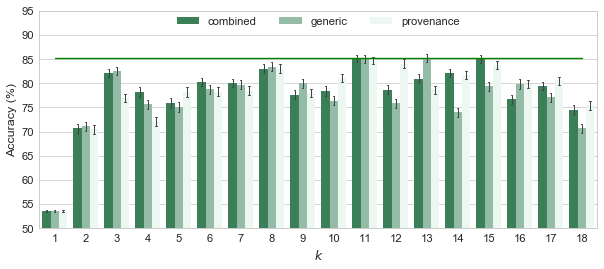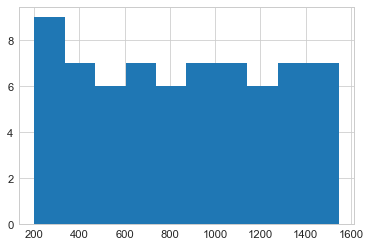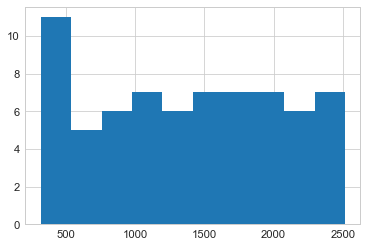# Application 3: RRG Chat Messages¶

Identifying instructions from chat messages in the Radiation Response Game

• Goal: To determine if the provenance network analytics method can identify instructions from the provenance of a chat messages.
• Classification labels: $\mathcal{L} = \left\{ \textit{instruction}, \textit{other} \right\}$.
• Training data: 69 chat messages manually categorised by HCI researchers.

The datasets from this application are provided in the folder rrg. Each CSV file, depgraphs-$k$ .csv with $k = 1 \ldots 18$, is a table whose rows correspond to individual chat messages in RRG:

• First column: the identifier of the chat message
• label: the manual classification of the message (e.g., instruction, information, requests, etc.)
• The remaining columns provide the provenance network metrics calculated from the dependency provenance graph of the message to the depth of $k$.
In :
import pandas as pd

In :
filepath = lambda k: "rrg/depgraphs-%d.csv" % k

In :
# An example of reading the data file
df = pd.read_csv(filepath(5), index_col=0)

Out:
label entities agents activities nodes edges diameter assortativity acc acc_e ... mfd_e_a mfd_e_ag mfd_a_e mfd_a_a mfd_a_ag mfd_ag_e mfd_ag_a mfd_ag_ag mfd_der powerlaw_alpha
21 requests 53 0 1 54 63 6 0.197008 0.393939 0.090909 ... 4 0 4 0 0 0 0 0 5 -1.0
20 commissives 57 0 3 60 84 7 0.046717 0.403367 0.105051 ... 5 0 5 0 0 0 0 0 5 -1.0
23 assertives 62 0 2 64 84 7 0.191105 0.393939 0.090909 ... 5 0 3 0 0 0 0 0 6 -1.0
25 instruction 55 0 2 57 77 6 0.128594 0.393939 0.090909 ... 5 0 5 4 0 0 0 0 5 -1.0
24 instruction 52 0 1 53 62 7 0.175250 0.394949 0.092424 ... 4 0 5 0 0 0 0 0 5 -1.0

5 rows × 23 columns

## Labelling data¶

Since we are only interested in the instruction messages, we categorise the data entity into two sets: instruction and other.

Note: This section is just an example to show the data transformation to be applied on each dataset.

In :
label = lambda l: 'other' if l != 'instruction' else l

In :
df.label = df.label.apply(label).astype('category')

Out:
label entities agents activities nodes edges diameter assortativity acc acc_e ... mfd_e_a mfd_e_ag mfd_a_e mfd_a_a mfd_a_ag mfd_ag_e mfd_ag_a mfd_ag_ag mfd_der powerlaw_alpha
21 other 53 0 1 54 63 6 0.197008 0.393939 0.090909 ... 4 0 4 0 0 0 0 0 5 -1.0
20 other 57 0 3 60 84 7 0.046717 0.403367 0.105051 ... 5 0 5 0 0 0 0 0 5 -1.0
23 other 62 0 2 64 84 7 0.191105 0.393939 0.090909 ... 5 0 3 0 0 0 0 0 6 -1.0
25 instruction 55 0 2 57 77 6 0.128594 0.393939 0.090909 ... 5 0 5 4 0 0 0 0 5 -1.0
24 instruction 52 0 1 53 62 7 0.175250 0.394949 0.092424 ... 4 0 5 0 0 0 0 0 5 -1.0

5 rows × 23 columns

## Balancing data¶

This section explore the balance of the RRG datasets.

In :
# Examine the balance of the dataset
df.label.value_counts()

Out:
other          37
instruction    32
Name: label, dtype: int64

Since both labels have roughly the same number of data points, we decide not to balance the RRG datasets.

## Cross validation¶

We now run the cross validation tests on the 18 datasets ($k = 1 \ldots 18$) using all the features (combined), only the generic network metrics (generic), and only the provenance-specific network metrics (provenance). The folowing steps are applied to each dataset:

1. Read the dataset from the CSV file
2. Label the data (see above)
3. Carry out the cross validation test
4. Append the test result into results and the feature importance into importances

Please refer to Cross Validation Code.ipynb for the detailed description of the cross validation code.

In :
from analytics import test_classification

In :
results = pd.DataFrame()
importances = pd.DataFrame()
for k in range(1, 19):
df = pd.read_csv(filepath(k), index_col=0)
df.label = df.label.apply(label).astype('category')

res, imps = test_classification(df, n_iterations=1000, test_id=str(k))
res['$k$'] = k
imps['$k$'] = k

# storing the results and importance of features
results = results.append(res, ignore_index=True)
importances = importances.append(imps, ignore_index=True)

Accuracy: 53.57% ±0.2216 <-- 1-combined
Accuracy: 53.57% ±0.2216 <-- 1-generic
Accuracy: 53.57% ±0.2216 <-- 1-provenance
Accuracy: 70.69% ±0.9766 <-- 2-combined
Accuracy: 71.06% ±0.9238 <-- 2-generic
Accuracy: 70.44% ±0.9567 <-- 2-provenance
Accuracy: 82.12% ±0.8706 <-- 3-combined
Accuracy: 82.59% ±0.8471 <-- 3-generic
Accuracy: 76.97% ±0.8917 <-- 3-provenance
Accuracy: 78.14% ±0.9607 <-- 4-combined
Accuracy: 75.64% ±0.9324 <-- 4-generic
Accuracy: 72.01% ±0.9712 <-- 4-provenance
Accuracy: 75.94% ±1.0142 <-- 5-combined
Accuracy: 75.04% ±0.9833 <-- 5-generic
Accuracy: 78.20% ±0.9767 <-- 5-provenance
Accuracy: 80.32% ±0.8902 <-- 6-combined
Accuracy: 78.80% ±0.8886 <-- 6-generic
Accuracy: 78.28% ±0.9354 <-- 6-provenance
Accuracy: 80.04% ±0.9246 <-- 7-combined
Accuracy: 79.71% ±0.9206 <-- 7-generic
Accuracy: 78.41% ±0.9294 <-- 7-provenance
Accuracy: 83.04% ±0.8573 <-- 8-combined
Accuracy: 83.43% ±0.8413 <-- 8-generic
Accuracy: 83.01% ±0.8509 <-- 8-provenance
Accuracy: 77.65% ±0.9467 <-- 9-combined
Accuracy: 80.00% ±0.9303 <-- 9-generic
Accuracy: 77.95% ±0.9707 <-- 9-provenance
Accuracy: 78.41% ±0.9444 <-- 10-combined
Accuracy: 76.35% ±0.9573 <-- 10-generic
Accuracy: 81.06% ±0.8990 <-- 10-provenance
Accuracy: 85.13% ±0.7883 <-- 11-combined
Accuracy: 85.11% ±0.8229 <-- 11-generic
Accuracy: 84.68% ±0.7948 <-- 11-provenance
Accuracy: 78.68% ±0.9448 <-- 12-combined
Accuracy: 75.82% ±0.9552 <-- 12-generic
Accuracy: 84.07% ±0.9171 <-- 12-provenance
Accuracy: 80.92% ±0.8581 <-- 13-combined
Accuracy: 85.24% ±0.8555 <-- 13-generic
Accuracy: 78.61% ±0.9031 <-- 13-provenance
Accuracy: 82.06% ±0.8376 <-- 14-combined
Accuracy: 73.98% ±0.9360 <-- 14-generic
Accuracy: 81.76% ±0.8362 <-- 14-provenance
Accuracy: 85.13% ±0.8144 <-- 15-combined
Accuracy: 79.38% ±0.9295 <-- 15-generic
Accuracy: 83.82% ±0.8687 <-- 15-provenance
Accuracy: 76.70% ±0.9083 <-- 16-combined
Accuracy: 79.91% ±0.9507 <-- 16-generic
Accuracy: 79.81% ±0.8504 <-- 16-provenance
Accuracy: 79.35% ±0.8664 <-- 17-combined
Accuracy: 77.12% ±0.8863 <-- 17-generic
Accuracy: 80.59% ±0.8716 <-- 17-provenance
Accuracy: 74.42% ±0.9875 <-- 18-combined
Accuracy: 70.79% ±0.9429 <-- 18-generic
Accuracy: 75.42% ±0.9804 <-- 18-provenance


Optionally, we can save the test results to save time the next time we want to re-explore them:

In :
results.to_pickle("rrg/results.pkl")
importances.to_pickle("rrg/importances.pkl")


Next time, we can reload the results as follows:

In :
import pandas as pd
results.shape, importances.shape

Out:
((54000, 3), (18000, 23))

## Charting the resutls¶

In :
%matplotlib inline
import seaborn as sns
sns.set_style("whitegrid")
sns.set_context("paper", font_scale=1.4)


For this application, with the many configuration to chart, it is difficult to determine which configuration yields the best accuracy from a figure. Instead, we determine this from the data. We group the performance of all classifiers by the set of metrics they used and the $k$ value; then, we calculate the mean accuracy of those groups.

In :
results['Accuracy'] = results['Accuracy'] * 100  # converting accuracy values to percent

In :
# define a function to calculate the mean and its confidence interval from a group of values
import scipy.stats as st
def calc_means_ci(group):
mean = group.mean()
ci_low, ci_high = st.t.interval(0.95, group.size - 1, loc=mean, scale=st.sem(group))
return pd.Series({
'mean': mean,
'ci_low': ci_low,
'ci_high': ci_high
})

In :
accuracy_by_metrics_k = results.groupby(["Metrics", "$k$"])  # grouping results by metrics sets and k

In :
# Calculate the means and the confidence intervals over the grouped data (using the calc_means_ci function above)
results_means_ci = accuracy_by_metrics_k.Accuracy.apply(calc_means_ci).unstack()
results_means_ci = results_means_ci[['mean', 'ci_low', 'ci_high']]  # reorder the column
results_means_ci

Out:
mean ci_low ci_high
Metrics $k$
combined 1 53.571429 53.349694 53.793163
2 70.694643 69.717504 71.671781
3 82.123810 81.252813 82.994806
4 78.135119 77.173957 79.096281
5 75.938095 74.923410 76.952781
6 80.321429 79.430772 81.212085
7 80.044048 79.118974 80.969121
8 83.039881 82.182169 83.897593
9 77.651786 76.704650 78.598922
10 78.414286 77.469403 79.359168
11 85.133333 84.344666 85.922001
12 78.677381 77.732121 79.622641
13 80.919048 80.060477 81.777618
14 82.057143 81.219102 82.895184
15 85.129762 84.314906 85.944618
16 76.697024 75.788237 77.605811
17 79.345238 78.478390 80.212086
18 74.423214 73.435232 75.411197
generic 1 53.571429 53.349694 53.793163
2 71.056548 70.132292 71.980803
3 82.589286 81.741715 83.436857
4 75.643452 74.710589 76.576315
5 75.035714 74.051876 76.019553
6 78.804762 77.915717 79.693807
7 79.705357 78.784296 80.626418
8 83.432143 82.590400 84.273886
9 80.003571 79.072804 80.934339
10 76.353571 75.395842 77.311301
11 85.112500 84.289158 85.935842
12 75.823214 74.867505 76.778923
13 85.239286 84.383318 86.095253
14 73.975000 73.038561 74.911439
15 79.379167 78.449196 80.309137
16 79.908333 78.957141 80.859525
17 77.121429 76.234707 78.008150
18 70.788690 69.845326 71.732055
provenance 1 53.571429 53.349694 53.793163
2 70.435119 69.477929 71.392309
3 76.973810 76.081626 77.865993
4 72.008333 71.036694 72.979972
5 78.201190 77.223992 79.178389
6 78.280357 77.344481 79.216234
7 78.414286 77.484401 79.344170
8 83.013095 82.161806 83.864384
9 77.951786 76.980571 78.923000
10 81.062500 80.163019 81.961981
11 84.680357 83.885165 85.475549
12 84.067857 83.150331 84.985383
13 78.607738 77.704217 79.511260
14 81.760714 80.924072 82.597356
15 83.820833 82.951727 84.689939
16 79.811905 78.961087 80.662722
17 80.589286 79.717217 81.461355
18 75.419048 74.438139 76.399957

Next, we sort the mean accuracy values in each metrics sets and find $k$ value that yields the highest accuracy for each set of metrics (i.e. combined, generic, and provenance).

In :
# Looking at only the means in each set of metrics
results_means_ci['mean'].unstack()

Out:
$k$ 1 2 3 4 5 6 7 8 9 10 11 12 13 14 15 16 17 18
Metrics
combined 53.571429 70.694643 82.123810 78.135119 75.938095 80.321429 80.044048 83.039881 77.651786 78.414286 85.133333 78.677381 80.919048 82.057143 85.129762 76.697024 79.345238 74.423214
generic 53.571429 71.056548 82.589286 75.643452 75.035714 78.804762 79.705357 83.432143 80.003571 76.353571 85.112500 75.823214 85.239286 73.975000 79.379167 79.908333 77.121429 70.788690
provenance 53.571429 70.435119 76.973810 72.008333 78.201190 78.280357 78.414286 83.013095 77.951786 81.062500 84.680357 84.067857 78.607738 81.760714 83.820833 79.811905 80.589286 75.419048
In :
# Finding the highest accuracy value in each row (i.e. each set of metrics)
highest_accuracy_configurations = [
(row_name, row.sort_values(ascending=False)[:1].index.get_values())  # the index (i.e. k value) of the highest accuracy (i.e. first one)
for row_name, row in results_means_ci['mean'].unstack().iterrows()
]
highest_accuracy_configurations

Out:
[('combined', 11), ('generic', 13), ('provenance', 11)]
In :
results_means_ci.loc[highest_accuracy_configurations, :]

Out:
mean ci_low ci_high
Metrics $k$
combined 11 85.133333 84.344666 85.922001
generic 13 85.239286 84.383318 86.095253
provenance 11 84.680357 83.885165 85.475549

The results above shows that $k = 13$ - generic yields the highest accuracy level: 85.24%. Using all the metrics or only the provenance-specific metrics yield comparable levels of accuracy (in the confidence interval of the highest accuracy) with $k = 11$.

For a visual comparison of all the configurations tested, we chart their accuracy next.

In :
pal = sns.light_palette("seagreen", n_colors=3, reverse=True)
plot = sns.barplot(x="$k$", y="Accuracy", hue='Metrics', palette=pal, errwidth=1, capsize=0.04, data=results)
plot.figure.set_size_inches((10, 4))
plot.legend(loc='upper center', bbox_to_anchor=(0.5, 1.02), ncol=3)
plot.set_ylabel('Accuracy (%)')
plot.set_ylim(50, 95)

# drawing a line at the highest accuracy for visual comparison between configurations
highest_accuracy = results_means_ci['mean'].max()
plot.axes.plot([0, 17], [highest_accuracy, highest_accuracy], 'g')
highest_accuracy

Out:
85.239285714285714The chart shows that the configurations yield the highest accuracy are: $k = 11$ - combined/generic/provenance, $k = 13$ - generic, and $k = 15$ - combined. The accuracy level seems to decrease with $k > 15$.

Saving the chart above to Fig6.eps to be included in the paper:

In :
plot.figure.savefig("figures/Fig6.eps")


## Analysing the importance of features¶

In this section, we explore the relevance of each features in classifying messages in RRG. To do so, we analyse the feature importance values provided by the decision tree training done above - the importances data frame.

In :
# Rename the columns with Math notation for consistency with the metrics symbols in the paper
feature_name_maths_mapping = {
"entities": "$n_e$", "agents": "$n_{ag}$", "activities": "$n_a$", "nodes": "$n$", "edges": "$e$",
"diameter": "$d$", "assortativity": "$r$", "acc": "$\\mathsf{ACC}$",
"acc_e": "$\\mathsf{ACC}_e$",  "acc_a": "$\\mathsf{ACC}_a$",  "acc_ag": "$\\mathsf{ACC}_{ag}$",
"mfd_e_e": "$\\mathrm{mfd}_{e \\rightarrow e}$", "mfd_e_a": "$\\mathrm{mfd}_{e \\rightarrow a}$",
"mfd_e_ag": "$\\mathrm{mfd}_{e \\rightarrow ag}$", "mfd_a_e": "$\\mathrm{mfd}_{a \\rightarrow e}$",
"mfd_a_a": "$\\mathrm{mfd}_{a \\rightarrow a}$", "mfd_a_ag": "$\\mathrm{mfd}_{a \\rightarrow ag}$",
"mfd_ag_e": "$\\mathrm{mfd}_{ag \\rightarrow e}$", "mfd_ag_a": "$\\mathrm{mfd}_{ag \\rightarrow a}$",
"mfd_ag_ag": "$\\mathrm{mfd}_{ag \\rightarrow ag}$", "mfd_der": "$\\mathrm{mfd}_\\mathit{der}$", "powerlaw_alpha": "$\\alpha$"
}
importances.rename(columns=feature_name_maths_mapping, inplace=True)

In :
grouped =importances.groupby("$k$")  # Grouping the importance values by k

In :
# Calculate the mean importance of each feature for each data type
imp_means = grouped.mean()

In :
three_most_relevant_metrics = pd.DataFrame(
{row_name: row.sort_values(ascending=False)[:3].index.get_values()  # three highest importance values in each row
for row_name, row in imp_means.iterrows()
}
)
three_most_relevant_metrics

Out:
1 2 3 4 5 6 7 8 9 10 11 12 13 14 15 16 17 18
0 $\alpha$ $e$ $e$ $e$ $n_e$ $n$ $n_e$ $n$ $e$ $n_e$ $n_e$ $e$ $e$ $\mathrm{mfd}_{e \rightarrow a}$ $e$ $n_e$ $\mathrm{mfd}_{a \rightarrow a}$ $\mathrm{mfd}_{a \rightarrow a}$
1 $\mathrm{mfd}_\mathit{der}$ $r$ $n_e$ $n_e$ $n$ $\mathrm{mfd}_{a \rightarrow a}$ $e$ $n_e$ $\mathrm{mfd}_{e \rightarrow a}$ $e$ $\mathsf{ACC}_e$ $\mathrm{mfd}_{a \rightarrow e}$ $\mathrm{mfd}_{e \rightarrow a}$ $e$ $\mathrm{mfd}_{e \rightarrow a}$ $\mathrm{mfd}_{e \rightarrow a}$ $\mathrm{mfd}_{e \rightarrow a}$ $\mathrm{mfd}_{e \rightarrow a}$
2 $n_{ag}$ $n_a$ $\mathsf{ACC}$ $d$ $e$ $n_e$ $\mathsf{ACC}$ $e$ $r$ $n$ $\mathsf{ACC}$ $\mathrm{mfd}_{a \rightarrow a}$ $\mathsf{ACC}$ $n_e$ $n_e$ $\mathsf{ACC}_e$ $e$ $\mathsf{ACC}_e$

The table above shows the most important metrics as reported by the decision tree classifiers during their training for each value of $k$.

Apart from $k = 1$, whose performance is no better than the random baseline, we count the occurences of the most relevant metrics in cases where $k \geq 2$ to find the most common metrics in the table above.

In :
metrics_occurrences = three_most_relevant_metrics.loc[:,2:].apply(pd.value_counts, axis=1).fillna(0)  # excluding k = 1
metrics_occurrences

Out:
$\mathrm{mfd}_{a \rightarrow a}$ $\mathrm{mfd}_{a \rightarrow e}$ $\mathrm{mfd}_{e \rightarrow a}$ $\mathsf{ACC}$ $\mathsf{ACC}_e$ $d$ $e$ $n$ $n_a$ $n_e$ $r$
0 2.0 0.0 1.0 0.0 0.0 0.0 7.0 2.0 0.0 5.0 0.0
1 1.0 1.0 6.0 0.0 1.0 0.0 3.0 1.0 0.0 3.0 1.0
2 1.0 0.0 0.0 4.0 2.0 1.0 3.0 1.0 1.0 3.0 1.0
In :
# sorting the sum of the metrics occurences
pd.DataFrame(metrics_occurrences.sum().sort_values(ascending=False), columns=['occurences'])

Out:
occurences
$e$ 13.0
$n_e$ 11.0
$\mathrm{mfd}_{e \rightarrow a}$ 7.0
$n$ 4.0
$\mathsf{ACC}$ 4.0
$\mathrm{mfd}_{a \rightarrow a}$ 4.0
$\mathsf{ACC}_e$ 3.0
$r$ 2.0
$n_a$ 1.0
$d$ 1.0
$\mathrm{mfd}_{a \rightarrow e}$ 1.0

As shown above, the number of edges $e$, the number of entities $n_e$, and the maximum finite distance between entities and activities $\mathrm{mfd}_{e \rightarrow a}$ are the most common metrics in the table of the most relevant metrics.

## Classification using full dependency graphs (Extra)¶

In this extra experiement, we tested run the same experiment as above but on the full dependency graphs of messages (similar to the experiements in Application 2), i.e. without restricting a dependency graph to $k$ edges away from a message entity. The provenance network metrics of those dependency graphs are provided in rrg/depgraphs.csv, which has the same format as the other CSV files provided in this application.

In :
# Reading the data
df = pd.read_csv("rrg/depgraphs.csv", index_col=0)
# Generate the label for classification
df.label = df.label.apply(label).astype('category')

In :
res, imps = test_classification(df, n_iterations=1000)

Accuracy: 65.49% ±1.0807 <-- combined
Accuracy: 60.76% ±1.1250 <-- generic
Accuracy: 64.91% ±1.0958 <-- provenance


Results: The above accuracy levels are very low (compared with the 50% baseline accuracy of random selection between two labels), indicating that the provenance network metrics of full dependency graphs of RRG messages do not correlate well with the nature of the messages.

The reason for this is that a RRG provenance graph captures all the activities in a game which are all connected. As a RRG provenance graph evolves linearly along the lifeline of a RRG game, the size of a dependency graph varies greatly depending on when in a game the message was sent; messages sent at the beginning of a game have significantly more (potential) dependants than those sent later in the game. This is shown in the histograms of the number of nodes and edges below. As a result, their network metrics also similarly vary (in another word, noisy) and are not a good predictor of the message type.

In :
df.nodes.hist()

Out:
<matplotlib.axes._subplots.AxesSubplot at 0x114b1ebe0>In :
df.edges.hist()

Out:
<matplotlib.axes._subplots.AxesSubplot at 0x115bbbda0>